# Box and Whisker Plots A Modern View of

• Slides: 14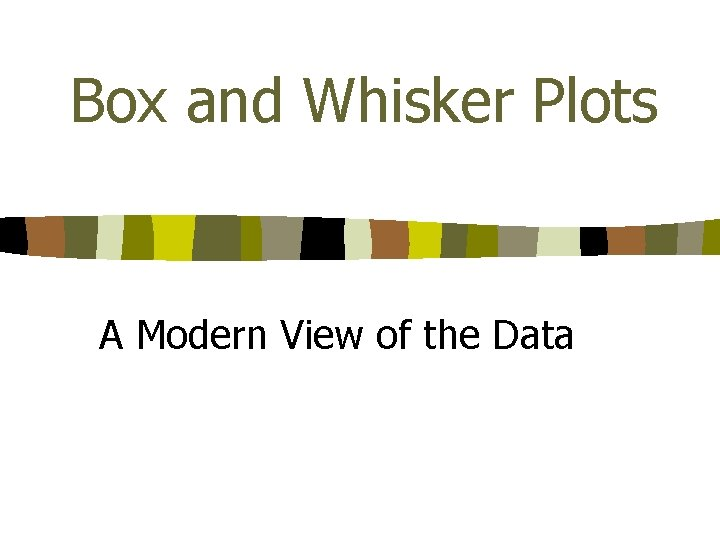Box and Whisker Plots A Modern View of the DataHistory Lesson n In 1977, John Tukey published an efficient method for displaying a five-number data summary. The graph is called a box plot (also known as a box and whisker plot) and summarizes the following statistical measures: qmedian qupper and lower quartiles qminimum and maximum data values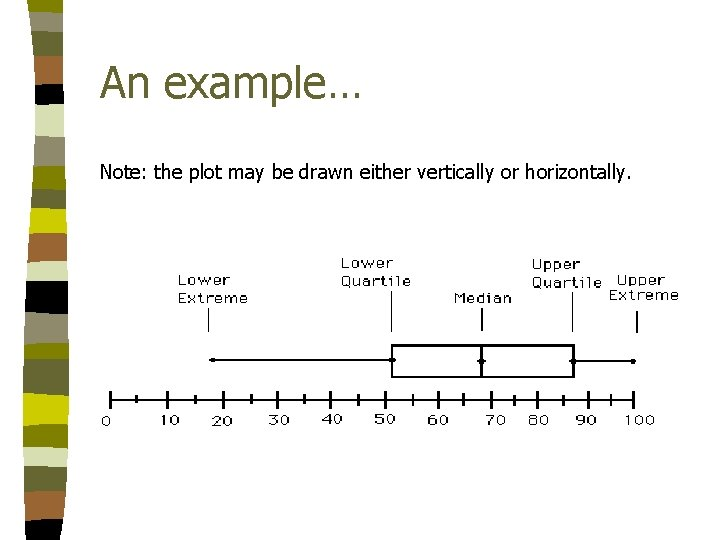An example… Note: the plot may be drawn either vertically or horizontally.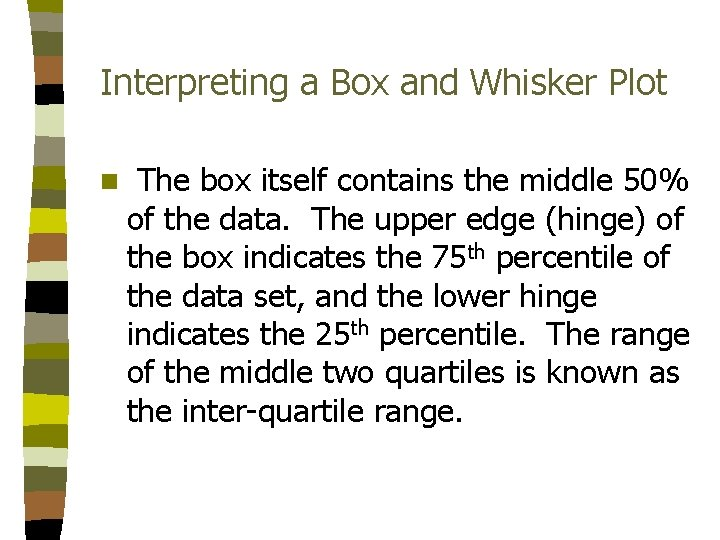Interpreting a Box and Whisker Plot n The box itself contains the middle 50% of the data. The upper edge (hinge) of the box indicates the 75 th percentile of the data set, and the lower hinge indicates the 25 th percentile. The range of the middle two quartiles is known as the inter-quartile range.Interpreting a Box and Whisker Plot (continued) n The line in the box indicates the median value of the data. n If the median line within the box is not equidistant from the hinges, then the data is skewed.Interpreting a Box and Whisker Plot (continued) n The ends of the vertical lines or “whiskers” indicate the minimum and maximum values, unless outliers are present in which case the whiskers extend to a maximum of 1. 5 times the inter-quartile range. n The points outside the ends of the “whiskers” are outliers or suspected outliers.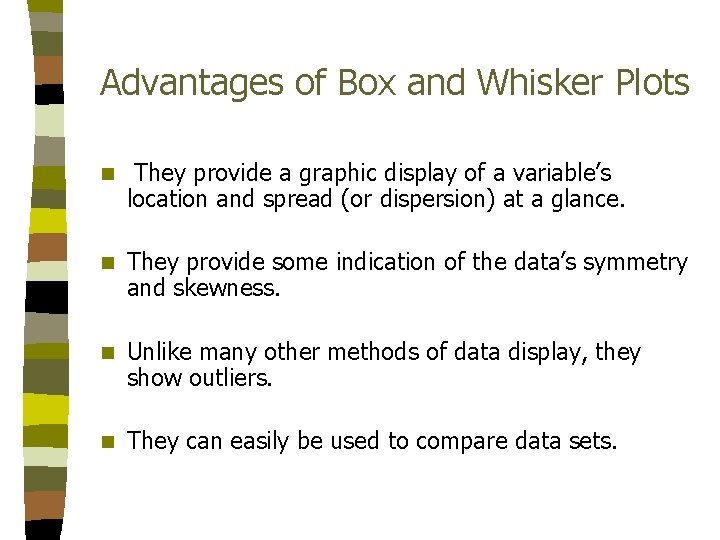Advantages of Box and Whisker Plots n They provide a graphic display of a variable’s location and spread (or dispersion) at a glance. n They provide some indication of the data’s symmetry and skewness. n Unlike many other methods of data display, they show outliers. n They can easily be used to compare data sets.Disadvantages of Box and Whisker Plots n They tend to emphasize the tails of a distribution, which are the least certain points in the data set. n They hide many details of the distribution.Constructing a box-andwhisker plot: n The data: Math test scores 80, 75, 90, 95, 65, 80, 85, 70, 100 n Write the data in numerical order and find the first quartile, the median, the third quartile, the smallest value and the largest value.Constructing a box-andwhisker plot: n median = 80 first quartile = 70 third quartile = 90 smallest value = 65 largest value = 100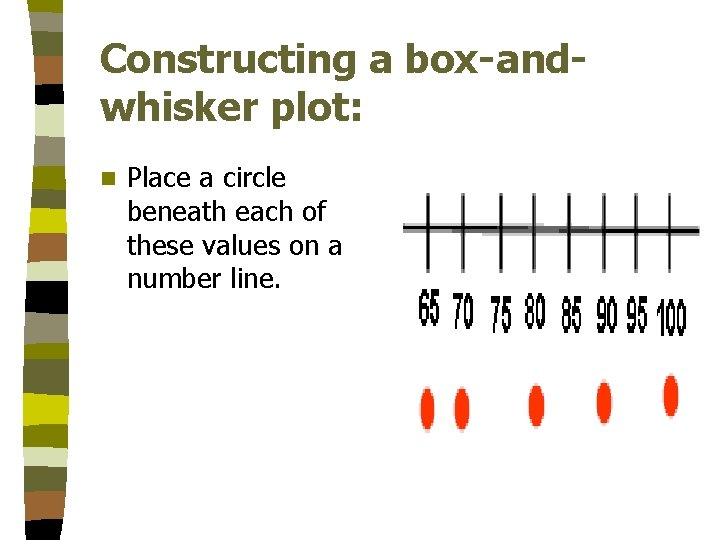Constructing a box-andwhisker plot: n Place a circle beneath each of these values on a number line.Constructing a box-andwhisker plot: n Draw a box with ends through the points for the first and third quartiles. Then draw a vertical line through the box at the median point. Now, draw the whiskers (or lines) from each end of the box to the smallest and largest values.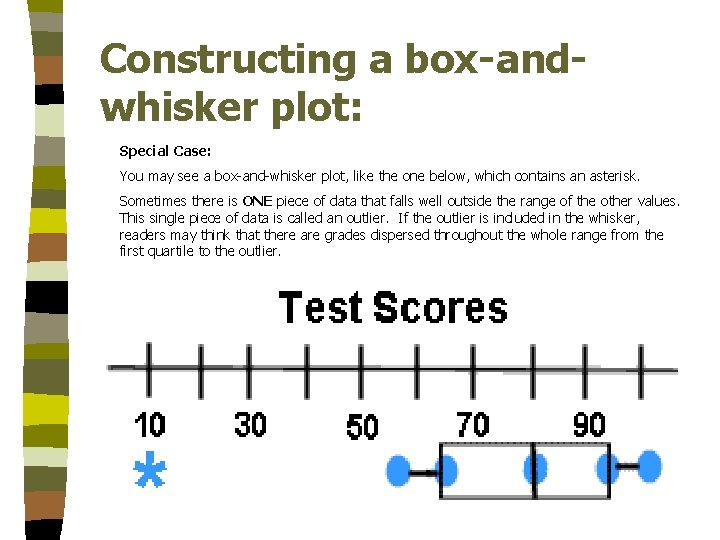Constructing a box-andwhisker plot: Special Case: You may see a box-and-whisker plot, like the one below, which contains an asterisk. Sometimes there is ONE piece of data that falls well outside the range of the other values. This single piece of data is called an outlier. If the outlier is included in the whisker, readers may think that there are grades dispersed throughout the whole range from the first quartile to the outlier.Questions? ? ?